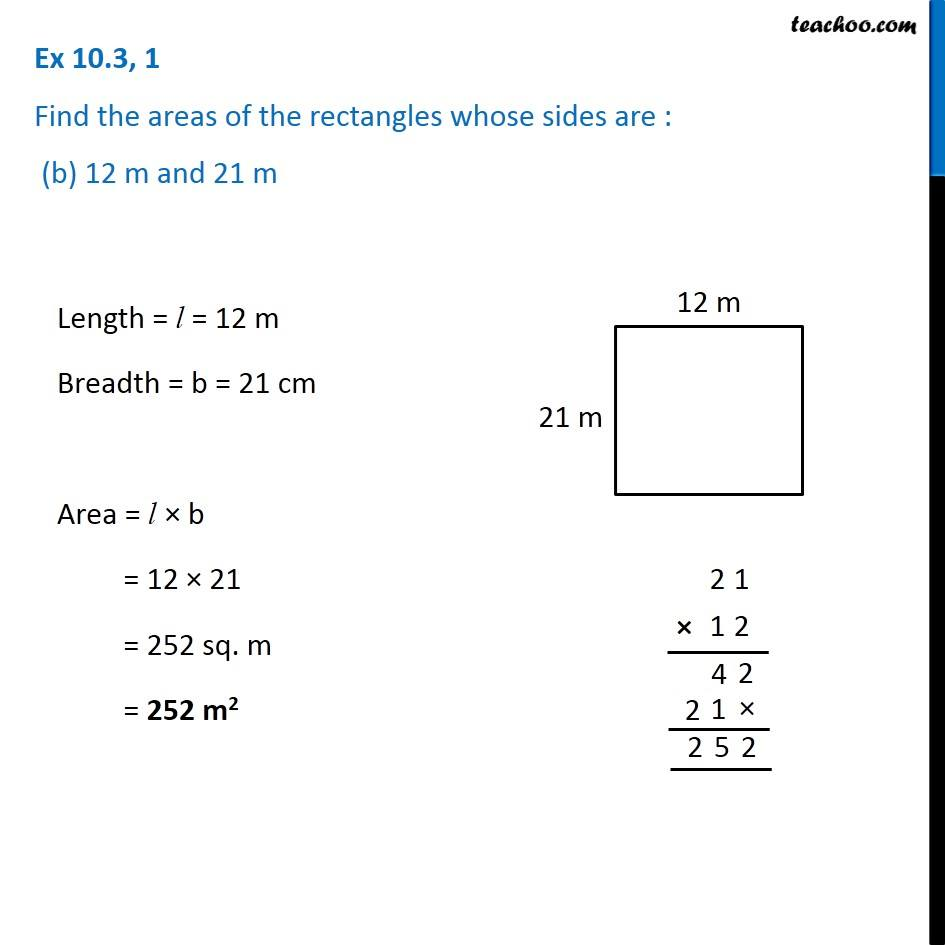1. Chapter 10 Class 6 Mensuration
2. Serial order wise
3. Ex 10.3

Transcript

Ex 10.3, 1 Find the areas of the rectangles whose sides are : (b) 12 m and 21 m Length = l = 12 m Breadth = b = 21 cm Area = l × b = 12 × 21 = 252 sq. m = 252 m2